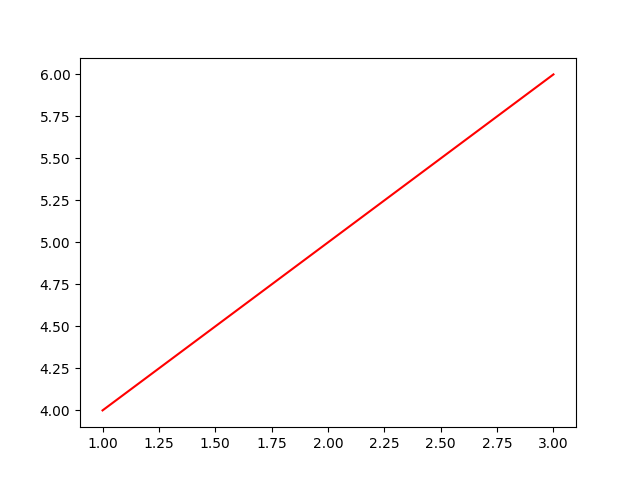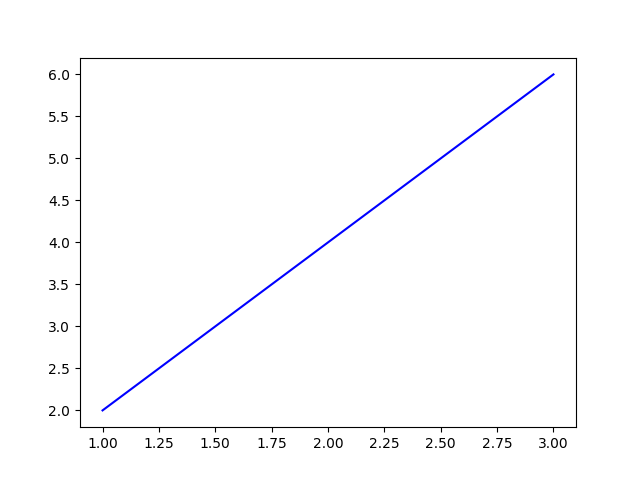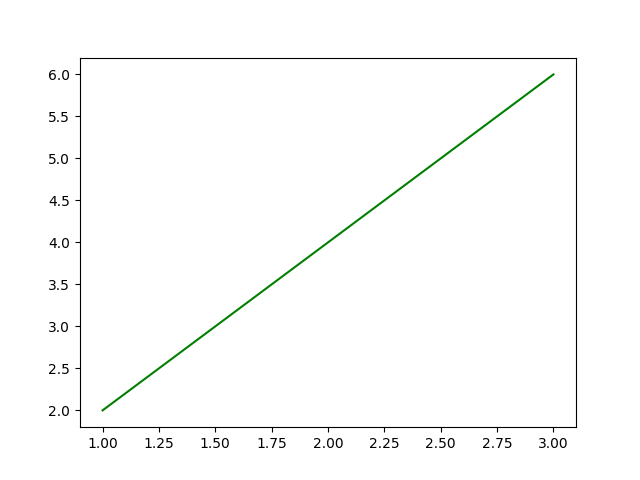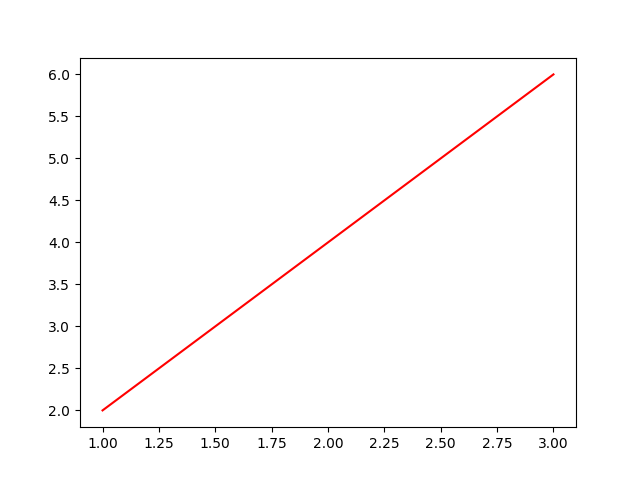# Make a plot, save, and show it (with and without blocking)¶

1. Regular plot show and save

2. Same but interactive and iterative (plt.ion() -> child threads, multi figs)

3. Use Bokeh to do some magic in a browser and draw connection to REST endpoint

for dashboard. Basically mention how we’ll likely use bokeh for graphs.

First let’s import our basic libraries

```import numpy as np
import matplotlib.pyplot as plt
import imagepypelines as ip
```

We’ll also need to set up a few blocks

```# A quick function which plots a line and styles it using plt.plot()'s kwargs
@ip.blockify(batch_type="all", void=True)  # plot=True -> sets batch type to "all" for similar UX? Not sure if worth aliasing
def line_plot(x, y, color="r"):
fig = plt.figure()
plt.plot(x, y, color=color)
plt.show()

line_plot_red = line_plot.tweak(color='r')
line_plot_green = line_plot.tweak(color='g')
line_plot_blue = line_plot.tweak(color='b')

"y": ip.Input(),
"red": (line_plot_red, "x", "y"),
"green": (line_plot_green, "x", "y"),
"blue": (line_plot_blue, "x", "y")
}

"y": ip.Input(),
"red": (line_plot.tweak(color='r'), "x", "y"),
"green": (line_plot.tweak(color='g'), "x", "y"),
"blue": (line_plot.tweak(color='b'), "x", "y")
}

# Normal functionality
line_plot([1,2,3], [4,5,6])

# Pipeline functionality - pre-defining separate instances
plotting([1,2,3],[2,4,6])

# Pipeline functionality - defining in place
plotting([1,2,3],[2,4,6])
```
•••••••Out:

```{'x': [1, 2, 3], 'y': [2, 4, 6], 'red': None, 'green': None, 'blue': None}
```

Total running time of the script: ( 0 minutes 0.635 seconds)

Gallery generated by Sphinx-Gallery